## Functional quantization of the Brownian motion and the Brownian bridge

Ifis a bi-measurable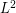stochastic process on, it can be considered as a random variable valued in the Hilbert space.

In , it is shown that in the Gaussian case, if the covariance function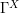is continuous, linear subspacesof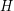spanned by-stationary codebooks correspond to principal components of, in other words, are spanned by eigenvectors of the covariance operator of.

Thus, the quantization consists first in exploiting the Karhunen-Loève decomposition. The discretization consists in truncating the decomposition at a fixed orderand to quantize the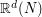-value Gaussian vector constituted of thefirst coordinates of the process on its Karhunen-Loève decomposition.

To reach optimal quantization, one has both to determine the optimal rank of truncation(the quantization dimension) and to determine the optimal-dimensional Gaussian quantizer corresponding to the first coordinates.

Formally, ifis a bi-measurableGaussian process, with a continuous covariance function, its Karhunen-Loève expansionwrites: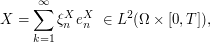whereis a sequence of independent Gaussian random variables.

The terms of the Karhunen-Loève decomposition are explicit for classical Gaussian processes (the standard Brownian motion, the Brownian bridge and the Ornstein-Uhlenbeck process).

• The Karhunen-Loève decomposition of the standard Brownian motiononis:• The Karhunen-Loève decomposition of the standard Brownian bridge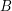onis: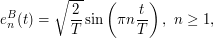In this case, the-dimensional random vector to be quantized is a Gaussian vector with diagonal variance-covariance matrix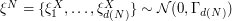withOptimal quantization of the Brownian motion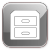brownian_optimal_grids.zip

The compressed folder brownian_optimal_grids.zip contains optimal quantization grids of the standard Brownian motion.

To get optimal quantization, the point now is to quantize the finite-dimensional Gaussian vector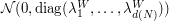optimally.

Hence, the method is the same as for the standard distribution except that the simulated Gaussian vector is not standard. For a given size, all possible dimensions are tested, and the one that yields the smaller quadratic distortion () is kept.

For a given size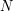, the text files are organized as follows. It presents in the form of a matrix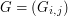with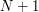rows and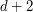columns.

• On row: Elementof the grid and its companion parameters. Consider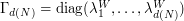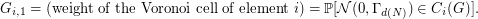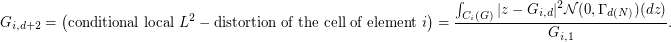• On last row: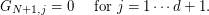In particular we can verify that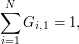and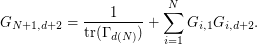For further details and further reading, let us refer to .

Product quantization of the Brownian motion and the Brownian bridge

An other way to get a good quantizer of a Gaussian process is Product Quantization. In practice,being settled, one determines the truncation thresholdof the decomposition and then,is approximated by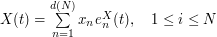whereis a quantizer of the-valued random vector.

The product quantization consists in choosing the quantizerof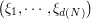as a Cartesian product of one dimensional quantization grids.

Thus, one replacesby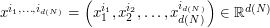where,are optimized quantizers of the-dimensional Gaussian distribution, of size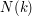, and where the valuesare such that. A database of optimal quadratic quantizers of the standard Gaussian distribution is available here.

After all, one has for a settled integerto determine among all its possible product decomposition the one that minimizes the distortion error.

In article , the optimal product decompositions are used to compute Asian option prices in a stochastic volatility model.RECORD_QF.TXTRECORD_QF_BB.TXT

The text file RECORD_QF.TXT contains optimal product decompositions for the standard Brownian motion of sizeto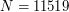.

The text file RECORD_QF_BB.TXT contains optimal product decompositions for the standard Brownian bridge of sizeto.

The both cases two first columns give, for a number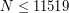, the value of the distortion of the optimal product quantization.

The following columns give the size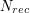of the best product quantizer for a maximum number of points of, and the corresponding distortion. At least, the corresponding optimal product decomposition is given.

### References

1. Harald Luschgy, and Gilles Pagès, Journal of Functional Analysis, vol. 196, no. 2: Academic Press, pp. 486–531, December, 2002.
2. Gilles Pagès, and Jacques Printems, Monte Carlo Methods and Appl., vol. 11, no. 11, pp. 407-446, 2005.